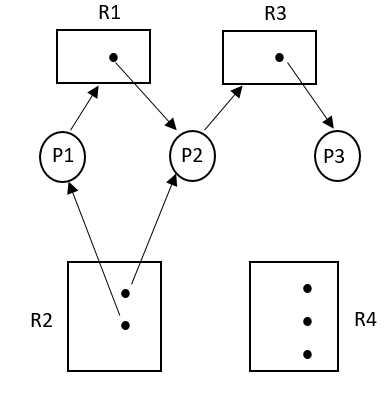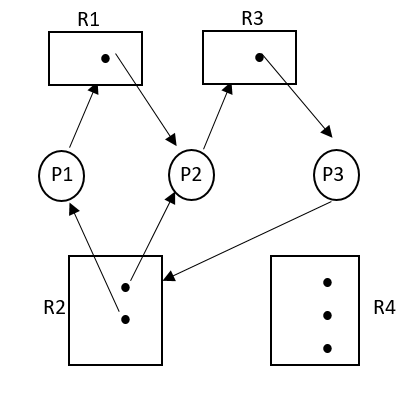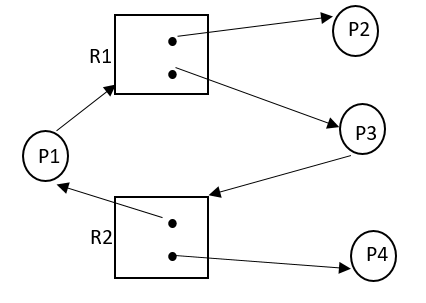# Introduction of Deadlock in Operating System

In this tutorial, we are going to learn about the deadlock in operating system, system model, deadlock characterization, resource allocation graph, etc.
Submitted by Sneha Dujaniya, on January 19, 2020

## 1.1 What exactly is a deadlock?

In a multiprogramming environment, there may be several processes with a finite number of resources. A process may request another resource while still holding some of the other resources with itself. If these requested resources are not available at the time, the process may enter into a waiting state. This waiting state may never end if the resources requested by this process are held by other waiting processes. This situation is called "Deadlock" where none of the processes are ready to release their resources and are also waiting indefinitely for other waiting processes to release their resources.

### 1.2 System Model

1. A system consists of a finite number of resources and these resources are partitioned into several types, each consisting of some number of identical instances.
2. Resource types include the printer, DVD drives, CPU cycles, files, etc. For example, if a system has two printers then the resource type Printer has two instances.
3. These instances may be identical or different. If the instances are similar, any instance can be assigned to the process, and if not, then the two printers need to be defined as separate resource classes.
4. A process must request the resource before using it and must release it after use.
5. A process may request as many resources as it requires for the completion of its task but it should not exceed the total number of resources present in the system.
6. To summarize, a process must utilize a resource in the following manner,
• Request: The process must request for the resource. If the request can't be granted immediately, it must wait to acquire the resource.
• Use: The process must use the resource after acquiring it.
• Release: The process must release the resource after using it.

There are four necessary conditions for a deadlock to occur. If any of the four conditions fail, then the condition is not called a deadlock and maybe recovered easily. The following are the necessary conditions,

1.3.1 Mutual Exclusion

At least one resource must be held in a non-sharable mode. This means that only one process can use the resource at a particular time. If any other process requests this non-sharable resource, then, it must wait for the process which is holding the resource to release it.

If we think clearly, this is a necessary condition for deadlock because if more than one process is allowed to use a resource at the same time, the deadlock will never occur and all the resources will carry out their tasks easily.

1.3.2 Hold and Wait

A process must be holding at least one resource and waiting to acquire other resources that are currently being held by other processes.

1.3.3 No preemption

The resources cannot be preempted. What this means is that one process cannot snatch a resource from another process that currently holds it. It can only be released voluntarily by the process holding it after its execution.

1.3.4 Circular Wait

A set {P0, P1, ..., Pn} of waiting process must exist such that P0 is waiting for a resource held by P1, P1 is waiting for a resource held by P2, …, Pn-1 is waiting for a resource held by Pn, and Pn is waiting for a resource held by P0.

The circular wait condition implies the hold and waits for condition, so these four conditions are not independent as we shall see in the next article.

### 1.4 Resource Allocation Graph

Resource allocation graphs are mainly used as methods for the detection of a deadlock. We will discuss this feature when we read about Deadlock Handling mechanisms.

1. Deadlock can be best represented in the terms of a directed graph called a system resource-allocation graph. The graph consists of a set of vertices V and a set of edges E.
2. The edges can be of two types, represented as Pi for a process i and Rj for a resource type j, where i, j are the integer values representing the number of processes and resource types respectively.
3. The vertices V are partitioned into two different types of nodes: P = {P1, P2, ..., Pn}, the set consisting of all the active processes in the system, and R = {R1, R2, ..., Rm}, the set consisting of all the resource types in the system.
4. A directed edge from process Pi to resource type Rj is denoted by PiRj. It shows that process Pi is requesting an instance of resource type Rj and is called a request edge.
5. A directed edge from resource type Rj to process Pi is denoted by RjPi. It shows that an instance of resource type Rj is allocated to process Pi and is called an assignment edge.
6. Pictorially, each process Pi is represented as a circle and each resource type Rj as a rectangle. Since there may be more than one instance of a resource, the instances are denoted by a dot within the rectangle.
7. When process Pi requests and instance of a resource type Rj, a request edge is inserted in the graph. When the request is fulfilled, the request edge is instantaneously transformed into an assignment edge. When the process completes its work, it releases the resource; as a result, the assignment edge is deleted.

Consider a few examples,

• The sets P, R, and E:
• P = {P1, P2, P3}
• R = {R1, R2, R3, R4}
• E = {P1 → R1, P2 → R3, R1 → P2, R2 → P2, R2 → P1, R3 → P3}
• Resource instances:
• One instance of resource type R1
• Two instances of resource type R2
• One instance of resource type R3
• Three instance of resource type R4

•Fig 1.1 Resource Allocation Graph
• Process states:
• Process P1 is holding an instance of resource type R2 and is waiting for an instance of resource type R1.
• Process P2 is holding an instance of R1 and an instance of R2 and is waiting for an instance of R3.
• Process P3 is holding an instance of R3.
• This resource allocation graph shows that there is no cycle in the graph. Therefore, no process in the system is deadlocked. If the graph does contain a cycle, then a deadlock may exist. This depends on two situations as follows.

IMPORTANT POINTS TO NOTE:

1. If each resource type has exactly one instance, then a cycle implies that a deadlock has occurred. Each process in the cycle is deadlocked. In this case, a cycle in the graph is both a necessary and sufficient condition for the existence of deadlock.
2. If each resource has several instances, then a cycle is not the necessary condition to determine that deadlock has occurred.
3. To illustrate this concept, we include a request edge from P3 → R2.Fig 1.2 Resource Allocation Graph (Cycle with deadlock)

Here, there are two cycles present in the system:

```    P1 → R1 → P2 → R3 → P3 → R2 → P1
P2 → R3 → P3 → R2 → P2
```

CONCLUSION: Processes P1, P2, and P3 are deadlocked.

Now, consider another graph where the following cycle exists:

```    P1 → R1 → P3 → R2 → P1
```

CONCLUSION: There is no deadlock. The process P4 may release its instance of resource type R2. That resource can then be allocated to P3, breaking the cycle.Fig 1.3 Cycle but no deadlock

To summarize: If a resource allocation graph does not have a cycle, then the system is not in a deadlocked state. If there is a cycle, then the system may or may not be in a deadlocked state. When there are multiple instances of a resource type, the presence of a deadlock is determined using Banker's Algorithm. We will study that in further articles.

References:

What's New

Top Interview Coding Problems/Challenges!

Languages: » C » C++ » C++ STL » Java » Data Structure » C#.Net » Android » Kotlin » SQL
Web Technologies: » PHP » Python » JavaScript » CSS » Ajax » Node.js » Web programming/HTML
Solved programs: » C » C++ » DS » Java » C#
Aptitude que. & ans.: » C » C++ » Java » DBMS
Interview que. & ans.: » C » Embedded C » Java » SEO » HR
CS Subjects: » CS Basics » O.S. » Networks » DBMS » Embedded Systems » Cloud Computing
» Machine learning » CS Organizations » Linux » DOS
More: » Articles » Puzzles » News/Updates